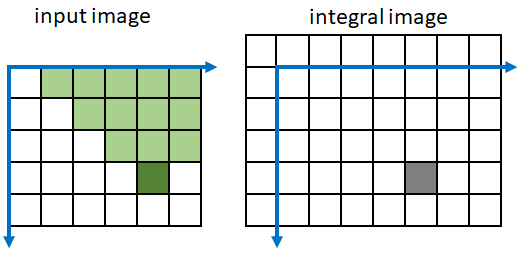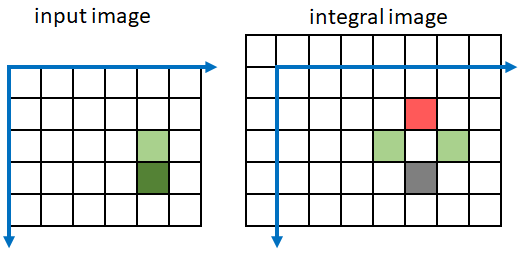## Mat 类

Mat 类可以用于表示多维的数组，数组的元素可以是单通道，也可以是多通道的。用图像来说明的话，黑白图片就是单通道的，因为每个像素只需要使用1个数值就能表示；彩色图片是3通道的，因为每个像素需要使用RGB3种三原色来表示。

### 创建和初始化

``````cv::Mat m;
m.create(3, 10, CV_32FC3);
``````

``````cv::Mat m;
m.create(3, [5, 5, 5], CV_32FC1);
``````

``````cv::Mat m;
m.create(3, 10, CV_32FC3);
m.setTo(Scalar(1.0f, 2.0f, 3.0f));
``````

``````Mat M(7,7,CV_32FC2,Scalar(1,3));
``````

``````u_char f = 0xff;
u_char dataArray = {
{0, 0, 0, 0, f, f, 0, 0, 0, 0},
{0, 0, 0, f, f, f, f, 0, 0, 0},
{0, 0, f, f, 0, 0, f, f, 0, 0},
}
``````

``````Mat mat = Mat(Size(10, 3), CV_8UC1, dataArray, sizeof(u_char) * 10);
``````

``````Mat::zeros(rows, cols, type);
Mat::ones(rows, cols, type);
Mat::eye(rows, cols, type);
``````

### 遍历与赋值

#### at<>()

OpenCV 的C++版深度使用了模板，at函数也不例外。

``````m.at<premitive type>(row, col)
``````

``````Mat m = Mat::eye(5, 3, CV_8UC2);
m.at<u_char>(1,2);
m.at<u_char>(1,2);
``````

#### 迭代器 Iterator

MatIterator<> 和 MatConstIterator<> 是 Mat类内置的迭代器。

``````    float a[] = {1.0f,2.0f,3.0f,4.0f,5.0f,6.0f,7.0f,8.0f,9.0f,10.0f,11.0f,12.0f,13.0f};
m = Mat(2, 2, CV_32FC3, a);
MatIterator_<Vec3f> m_begin = m.begin<Vec3f>();
MatIterator_<Vec3f> m_end = m.end<Vec3f>();
while (m_begin != m_end)
{
cout<< (*m_begin)<<", "<<(*m_begin)<<", "<<(*m_begin)<<endl;
(*m_begin) = 111;
m_begin++;
}
``````

begin和end方法中需要指定type，书中不知道是否是印刷错误还是老版本的问题，缺少了在v4.5版本中编译会出错。

#### ptr

ptr方法是搜索的时候看到的，在书中第4章并没有提及，其用法如下：

``````    for(int i = 0; i < m.rows; i ++) {
float *ptr = m.ptr<float>(i);
for (int j = 0; j < m.cols*m.channels(); j++)
{
cout<<"("<<i<<","<<j<<"):"<<(float)ptr[j]<<"  ";
}
cout<<endl;

}
``````

#### NAryMatIterator

Exercise4-1: iterate high dims mat

``````    //exercise4-1: iterate high dims mat
const int n_mat_size = 5;
const int n_mat_sz[] = {n_mat_size, n_mat_size, n_mat_size};
Mat n_mat(3, n_mat_sz, CV_32FC1);

RNG rng;
rng.fill(n_mat, RNG::UNIFORM, 0.f, 1.f);
const Mat* arrays[] = {&n_mat, 0};
Mat my_planes;
NAryMatIterator it(arrays, my_planes);

float s = 0.f;
int n = 0;
cout<<"nplanes:"<<it.nplanes<<endl<<"narrays:"<<it.narrays<<endl;

for (int p = 0; p < it.nplanes; p++, ++it)
{
cout<<it.planes<<endl;
cout<<"tmp:"<<sum(it.planes)<<endl;
s += sum(it.planes);
n ++;
}
cout<<"s:"<<s<<endl;
``````

exercise4-2: iterate multiple mats

``````    //exercise4-2: iterate multiple mats
const int n_mat_size = 5;
const int n_mat_sz[] = {n_mat_size, n_mat_size, n_mat_size};
Mat n_mat0(3, n_mat_sz, CV_32FC1);
Mat n_mat1(3, n_mat_sz, CV_32FC1);

RNG rng;
rng.fill(n_mat0, RNG::UNIFORM, 0.f, 1.f);
rng.fill(n_mat1, RNG::UNIFORM, 0.f, 1.f);

const Mat* arrays[] = {&n_mat0, &n_mat1, 0};
Mat my_planes;
NAryMatIterator it(arrays, my_planes);

float s= 0.f;
int n = 0;
cout<<"nplanes:"<<it.nplanes<<endl;
for(int p = 0; p < it.nplanes; p++, ++it) {
cout<<"plane:"<<it.planes<<endl;
cout<<"plane:"<<it.planes<<endl;
s+= sum(it.planes);
s+= sum(it.planes);
n++;
}
cout<<"n:"<<n<<endl;
``````

### Saturation Casting

saturate_cast<>是在做操作的时候，设置元素的取值范围，作用是防止溢出。比如对于uchar取值是在 0～128。如果数值小于0，则返回0；如果数值大于128，则返回128。

## SpartMat 类

SpartMat 的用法：

``````    int size[] = {10, 10};
SparseMat sm(2, size, CV_32F);
for (int i = 0; i < 10; i++)
{
int idx;
idx = size * rand();
idx = size * rand();
printf("%d, %d\n", idx, idx);

sm.ref<float>(idx) += 1.0f;
}

SparseMatConstIterator_<float> it       = sm.begin<float>();
SparseMatConstIterator_<float> it_end   = sm.end<float>();

for (; it != it_end; it++)
{
const SparseMat::Node* node = it.node();

printf("(%3d, %3d)  %f\n", node->idx, node->idx, *it);
}
``````

## 大型数组类型的模板结构

``````typedef Point_<float> Point2f;
``````

Point_ 就是通过 `template<typename _Tp> class Point_` 模板类声明的。

``````template<typename _Tp> class Mat_ : public Mat
``````

## 作业

### 作业1

1. 创建一个500x500 的区域，区域内单通道并初始化每个元素为0；
2. 输入数字，在区域内显示，每个数字大小 10 x 20；
3. 从左往右输入，到到区域右侧后，即使有输入也不再显示；
4. 支持删除和换行，还可以通过左右上下键进行编辑；
5. 输入某个键，支持将图像切换到彩色，每一个数字有其独特的颜色；

1. 如何显示数字；
2. 如何局部赋值；

``````u_char f = 0xff;

u_char twoArrays = {
{0, 0, 0, 0, f, f, 0, 0, 0, 0},
{0, 0, 0, f, f, f, f, 0, 0, 0},
{0, 0, f, f, 0, 0, f, f, 0, 0},
{0, f, f, 0, 0, 0, 0, f, f, 0},
{f, f, 0, 0, 0, 0, 0, 0, f, f},
{f, 0, 0, 0, 0, 0, 0, 0, f, f},
{0, 0, 0, 0, 0, 0, 0, f, f, 0},
{0, 0, 0, 0, 0, 0, 0, f, f, 0},
{0, 0, 0, 0, 0, 0, f, f, 0, 0},
{0, 0, 0, 0, 0, f, f, 0, 0, 0},
{0, 0, 0, 0, f, f, 0, 0, 0, 0},
{0, 0, 0, 0, f, f, 0, 0, 0, 0},
{0, 0, 0, f, f, 0, 0, 0, 0, 0},
{0, 0, 0, f, f, 0, 0, 0, 0, 0},
{0, 0, f, f, 0, 0, 0, 0, 0, 0},
{0, 0, f, f, 0, 0, 0, 0, 0, 0},
{0, f, f, 0, 0, 0, 0, 0, 0, 0},
{f, f, 0, 0, 0, 0, 0, 0, 0, 0},
{f, f, f, f, f, f, f, f, f, f},
{f, f, f, f, f, f, f, f, f, f}
};
Mat mat2 = Mat(Size(10, 20), CV_8UC1, twoArrays, sizeof(u_char) * 10);
``````

``````//i: row, j: col
mat2.copyTo(mat(Range(j * 20, j *20 + 20), Range((i - 1) * 10, (i - 1)*10 + 10)));
``````

``````    while (true)
{
auto keyPress = waitKey(20);
if (-1 != keyPress)
{
cout << "keyPress:" << keyPress << endl;
}
switch (keyPress) {
//
}
}
``````

### 作业2

#### 积分图像 Integral Image

``````1 2 3 4 5
6 7 8 9 10
``````

``````1 3  6  10 15
7 16 27 40 55
``````

``````1 3  6  10 15
7 16 ?
``````

``````x  x  x  x  x
x  x  C  B  x
x  x  A  ?
``````

``````    for(int i = 0; i < rows; i++) {
for (int j = 0; j < cols; j++) {
if(0 == i && 0 == j) {
mat_integral2.at<float>(i, j) = mat_uchar.at<uchar>(i, j);
}else if (0 == i && 0 != j) {
mat_integral2.at<float>(i, j) = mat_integral2.at<float>(i, j - 1) + mat_uchar.at<uchar>(i, j);
}else if (0 != i && 0 == j) {
mat_integral2.at<float>(i, j) = mat_integral2.at<float>(i - 1, j) + mat_uchar.at<uchar>(i, j);
}else {
mat_integral2.at<float>(i, j) = mat_integral2.at<float>(i, j - 1) + mat_integral2.at<float>(i - 1, j) - mat_integral2.at<float>(i - 1, j - 1) + mat_uchar.at<uchar>(i, j);
}
}
}
``````

``````    Mat mat_integral = Mat::zeros(Size(cols+1, rows+1), CV_32FC1);
for(int i = 1; i < rows + 1; i++) {
for (int j = 1; j < cols + 1; j++) {
mat_integral.at<float>(i, j) = mat_integral.at<float>(i, j - 1) + mat_integral.at<float>(i - 1, j) - mat_integral.at<float>(i - 1, j - 1) + mat_uchar.at<uchar>(i - 1, j - 1);
}
}
``````

OpenCV中的 API integral 的输入参数中也是传入 Size(cols+1, rows+1) 的目标 mat。

`Sum(ABCD) = I(C)-I(d)-I(b)+I(a)` 求得。

``````------------------------------------->x
|
|
|                        b
|              aA--------B
|               |        |
|               |        |
|              dD--------C
|
|
↓
y
``````

#### 旋转积分图像 Tiled Integral Image